## 9.4.8 Ellipses

Ellipsis (omission mark) commands are shown in Table 9.12. The amsmath package also provides: `\dotsc` for dots with commas, `\dotsb` for dots with binary operators/relations, `\dotsm` for multiplication dots, `\dotsi` for dots with integrals and `\dotso` for other dots, which can be used as replacements for `\ldots` and `\cdots`.

 `\vdots`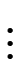`\cdots`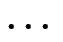`\dotsb`†`\dotsi`†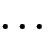`\dotsm`†`\ddots`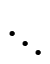`\ldots`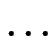`\dotsc`†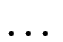`\dotso`†Example (Low Ellipsis):

This example uses the command `\forall` to produce the “for all” symbol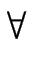, and it also uses \␣ (backslash space) to make a space before the for all symbol. The amsmath “dots with commas” ellipsis `\dotsc` is used rather than the standard `\ldots`:Example (Centred ellipsis):

This example uses the amsmath “dots with binary operators/relations” `\dotsb` instead of the standard `\cdots`:

\[
y = a_1 + a_2 + `\dotsb` + a_n
\]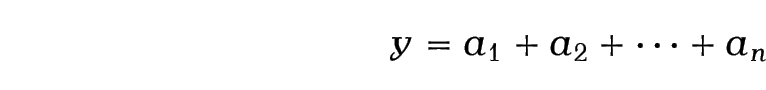Exercise 22: Maths: Fractions and Symbols

This exercise uses a fraction, a square root, subscripts, superscripts and symbols. Try to reproduce the following output: